# Pandas – Merge two dataframes with different columns

• Last Updated : 29 Oct, 2021

Pandas support three kinds of data structures. They are Series, Data Frame, and Panel. A Data frame is a two-dimensional data structure, Here data is stored in a tabular format which is in rows and columns. We can create a data frame in many ways.

Here we are creating a data frame using a list data structure in python.

## Python3

 `# import required module``import` `pandas`` ` `# assign data``l``=``[``"vignan"``,``"it"``,``"sravan"``,``"subbarao"``]`` ` `# create data frame``df ``=` `pandas.DataFrame(l)`` ` `# display dataframe``df`

Output: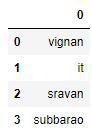Here in the above example, we created a data frame. Let’s merge the two data frames with different columns. It is possible to join the different columns is using concat() method.

Syntax: pandas.concat(objs: Union[Iterable[‘DataFrame’], Mapping[Label, ‘DataFrame’]], axis=’0′, join: str = “‘outer'”)

• DataFrame: It is dataframe name.
• Mapping: It refers to map the index and dataframe columns
• axis: 0 refers to the row axis and1 refers the column axis.
• join: Type of join.

Note: If the data frame column is matched. Then empty values are replaced by NaN values.

### Steps by step Approach:

• Open jupyter notebook
• Import necessary modules
• Create a data frame
• Perform operations
• Analyze the results.

Below are some examples based on the above approach:

Example 1

In this example, we are going to concatenate the marks of students based on colleges.

## Python3

 `# importing pandas module``import` `pandas as pd`` ` ` ` `# dictionary with list object in ``# values ie college details``details ``=` `{``    ``'Name'``: [``'Sravan'``, ``'Sai'``, ``'Mohan'``, ``'Ishitha'``],``    ``'College'``: [``'Vignan'``, ``'Vignan'``, ``'Vignan'``, ``'Vignan'``],``    ``'Physics'``: [``99``, ``76``, ``71``, ``93``],``    ``'Chemistry'``: [``97``, ``67``, ``65``, ``89``],``    ``'Data Science'``: [``93``, ``65``, ``65``, ``85``]``}`` ` ` ` `# converting to dataframe using DataFrame()``df ``=` `pd.DataFrame(details)`` ` ` ` `# print data frame``df`

Output: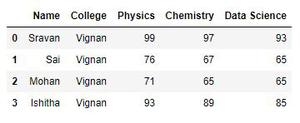## Python3

 `# creating another data``details1 ``=` `{``    ``'Name'``: [``'Harsha'``, ``'Saiteja'``, ``'abhilash'``, ``'harini'``],``    ``'College'``: [``'vvit'``, ``'vvit'``, ``'vvit'``, ``'vvit'``],``    ``'Physics'``: [``69``, ``76``, ``51``, ``43``],``    ``'Chemistry'``: [``67``, ``67``, ``55``, ``89``],``    ``'Maths'``: [``73``, ``65``, ``61``, ``85``]``}`` ` `# create dataframe``df1 ``=` `pd.DataFrame(details1)`` ` ` ` `# display dataframe``df1`

Output: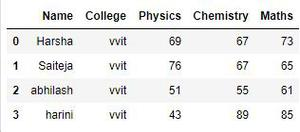## Python3

 `# concat dataframes``pd.concat([df, df1], axis``=``0``, ignore_index``=``True``)`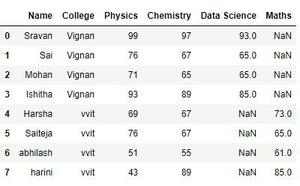## Python3

 `# concat when axis = 1``pd.concat([df, df1], axis``=``1``, ignore_index``=``True``)`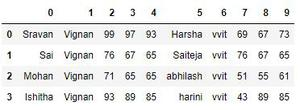Example 2:

Storing marks and subject details

## Python3

 `# Import pandas library``import` `pandas as pd`` ` `# initialize list of lists``data ``=` `[[``'sravan'``, ``98.00``], [``'jyothika'``, ``90.00``], [``'vijay'``, ``79.34``]]`` ` `# Create the pandas DataFrame``df ``=` `pd.DataFrame(data, columns``=``[``'Name'``, ``'Marks'``])`` ` `# print dataframe.``df`

Output: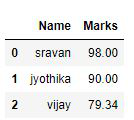## Python3

 `# initialize list of lists``data1 ``=` `[[``'Haseen'``, ``88.00``, ``5``], [``'ramya'``, ``54.00``, ``5``], [``'haritha'``, ``56.34``, ``4``]]`` ` `# Create the pandas DataFrame``df1 ``=` `pd.DataFrame(``    ``data1, columns``=``[``'Name'``, ``'Marks'``, ``'Total subjects registered'``])`` ` `# print dataframe.``df1`

Output: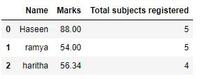## Python3

 `# concatenating data frame``pd.concat([df, df1], axis``=``0``, ignore_index``=``True``)`

Output: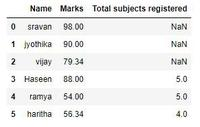My Personal Notes arrow_drop_up